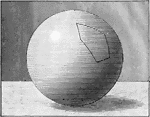### Spherical Polygon

Illustration of a spherical polygon.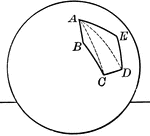### Spherical Polygon

Illustration of a spherical polygon.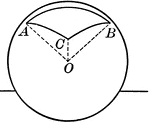### Spherical Pyramid

Illustration of a spherical pyramid.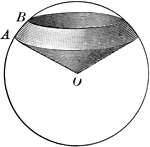### Spherical Sector

Illustration of a spherical sector.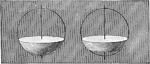### Spherical Segments

Illustration of a spherical segments.### Semicircle With Chords and Radii

Illustration of a semicircle with chords and radii.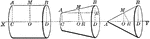### Solids of Revolution

Illustration of an isosceles spherical triangle.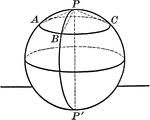### Sphere With Arcs and Poles

A sphere with arcs and poles. "The distance of all points in the circumference of a circle of a sphere…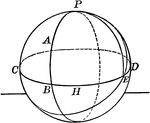### Arcs Drawn on Sphere

Illustration of a sphere with arcs drawn.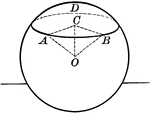### Circle Made by Plane Passing Through a Sphere

"Every section of a sphere made by a plane is a circle."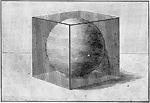### Sphere Inscribed in a Cube

Illustration of a sphere inscribed in a cube.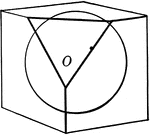### Sphere Inscribed in a Cube

Illustration of a sphere inscribed in a cube.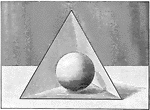### Sphere Inscribed in Tetrahedron

Illustration of a sphere inscribed in a tetrahedron.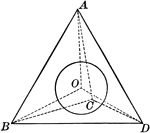### Sphere Inscribed in Tetrahedron

Illustration of a sphere inscribed in a tetrahedron.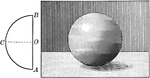### Sphere Made by Revolution of Semicircle

Illustration of a sphere generated by the revolution of a semicircle ACB about its diameter AB as an…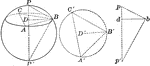### Material Sphere

Illustration of a material sphere that can be used to find its diameter.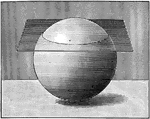### Plane Passing Through a Sphere

Illustration of a plane passing through a sphere.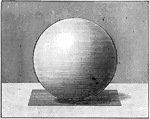### Sphere With Tangent Plane

Illustration of a sphere with a tangent plane. "A plane perpendicular to a radius at its extremity is…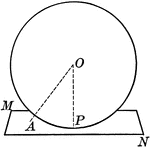### Sphere With Tangent Plane

Illustration of a sphere with a tangent plane. "A plane perpendicular to a radius at its extremity is…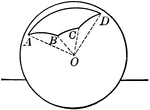### Polyhedral Angle in a Sphere

Illustration of a sphere with a polyhedral angle whose vertex is at the center of the sphere and whose…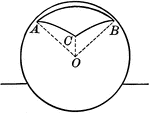### Polyhedral Angle in a Sphere

Illustration of a sphere with a polyhedral angle.### Sphere With Quadrants and Poles

A sphere with quadrants and poles. "A point on the surface of a sphere, which is at the distance of…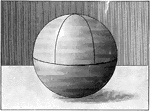A sphere with quadrants drawn on it.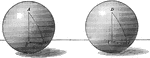### Equal Spheres With Triangles

Illustration of equal spheres with equal triangles.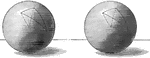### Equal Spheres With Triangles

Illustration of equal spheres with equilateral and equiangular triangles.### Intersecting Spheres

Illustration of two intersecting spheres. "The intersection of two spherical surfaces is the circumference…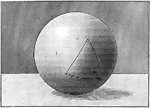### Isosceles Spherical Triangle

Illustration of an isosceles spherical triangle.### Isosceles Spherical Triangle

Illustration of an isosceles spherical triangle.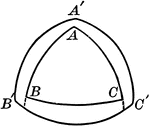### Polar and Spherical Triangles

Illustration of polar and spherical triangles.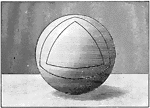### Polar Triangles in Sphere

Illustration of polar triangles.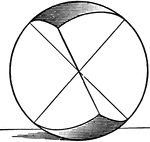### Symmetric Spherical Triangles

Illustration of symmetrical spherical triangles.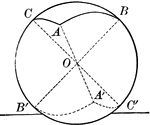### Symmetric Spherical Triangles

Illustration of symmetrical spherical triangles.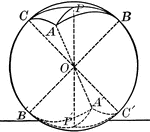### Symmetric Spherical Triangles

Illustration of symmetrical spherical triangles.